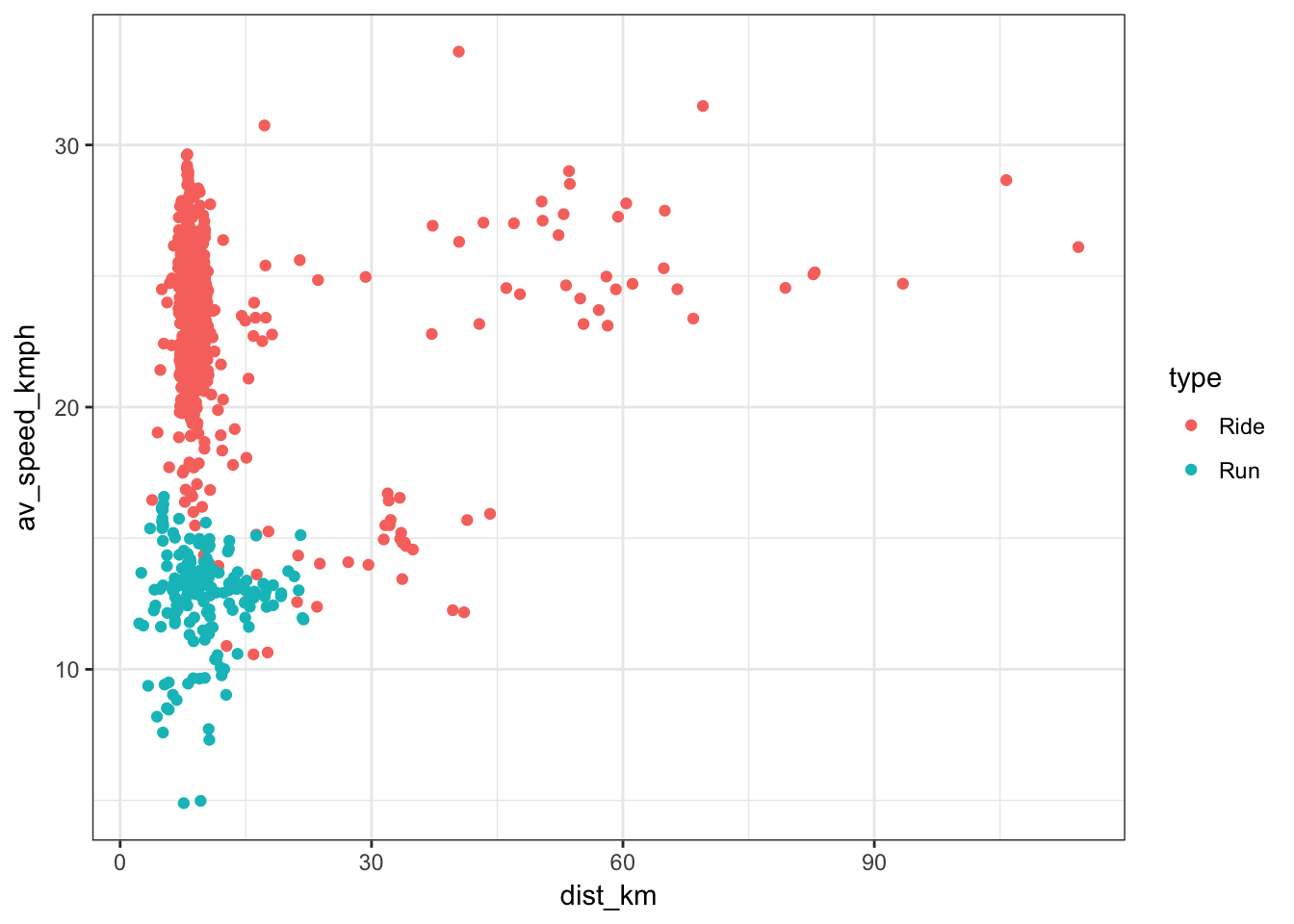For over a year now, I have been recording my daily commute by bike, as well as other recreational cycles and runs, on a widely used app called Strava. By accessing the Strava API, it is possible (and not all that hard, I used the steps described here: http://www.open-thoughts.com/2017/01/the-quantified-cyclist-analysing-strava-data-using-r/) to import data about these sessions to analyse in a program such as R. In addition, we can obtain the raw GPS data by downloading .gpx files directly from the Strava profile page, as described in this R package: https://github.com/marcusvolz/strava/ . In this post, I will explore some of these data.

First we need to combine two different data sets: the GPS data and the data from the strava API. These tell us different things but should be describing the same rides.

``````library(dplyr)
new_data <- gpx_data %>%
group_by(id) %>%
summarise(start_date = min(time) %>%
as.character %>%
gsub(' ', 'T', .) %>%
paste(.,'Z',sep='') ) %>%
left_join(strava_data,.,by=c('start_date')) %>%
left_join(gpx_data,.,by=c('id'))``````

Here is a summary of the combined dataset:

``str(new_data)``
``````## 'data.frame':    1729581 obs. of  22 variables:
##  \$ id                  : int  1 1 1 1 1 1 1 1 1 1 ...
##  \$ lat                 : num  51.8 51.8 51.8 51.8 51.8 ...
##  \$ lon                 : num  -1.26 -1.26 -1.26 -1.26 -1.26 ...
##  \$ ele                 : num  71.7 71.9 71.9 71.9 71.9 71.8 71.7 71.6 71.6 71.5 ...
##  \$ time                : POSIXct, format: "2016-02-13 09:31:30" "2016-02-13 09:32:02" ...
##  \$ dist_to_prev        : num  0 0.02392 0.00476 0.00781 0.00577 ...
##  \$ cumdist             : num  0 0.0239 0.0287 0.0365 0.0423 ...
##  \$ time_diff_to_prev   : num  0 32 1 2 2 2 2 2 2 1 ...
##  \$ cumtime             : num  0 32 33 35 37 39 41 43 45 46 ...
##  \$ name                : chr  "Red flag run" "Red flag run" "Red flag run" "Red flag run" ...
##  \$ commute             : logi  FALSE FALSE FALSE FALSE FALSE FALSE ...
##  \$ distance            : num  8111 8111 8111 8111 8111 ...
##  \$ athlete_count       : int  1 1 1 1 1 1 1 1 1 1 ...
##  \$ total_elevation_gain: num  15 15 15 15 15 15 15 15 15 15 ...
##  \$ elapsed_time        : int  3122 3122 3122 3122 3122 3122 3122 3122 3122 3122 ...
##  \$ type                : chr  "Run" "Run" "Run" "Run" ...
##  \$ kudos_count         : int  0 0 0 0 0 0 0 0 0 0 ...
##  \$ average_speed       : num  2.63 2.63 2.63 2.63 2.63 ...
##  \$ max_speed           : num  4.3 4.3 4.3 4.3 4.3 4.3 4.3 4.3 4.3 4.3 ...
##  \$ average_watts       : num  NA NA NA NA NA NA NA NA NA NA ...
##  \$ pr_count            : int  0 0 0 0 0 0 0 0 0 0 ...
##  \$ start_date          : chr  "2016-02-13T09:31:30Z" "2016-02-13T09:31:30Z" "2016-02-13T09:31:30Z" "2016-02-13T09:31:30Z" ...``````

First lets scale some of the features to give more familiar units, and pick out the runs and rides separately.

``````#process data to convert units
processed_data <- mutate(new_data,dist_km = distance/1000,av_speed_kmph = average_speed*3600/1000,time_mins = elapsed_time/60)``````

Visualising this data gives us a better idea of how it is structured. How different are my runs and rides? Can we tell them apart?

``````library(ggplot2)
grouped_by_id <- processed_data %>%
group_by(id) %>%
summarise(dist_km = unique(dist_km),
av_speed_kmph = unique(av_speed_kmph),
type = unique(type))
ggplot(data = grouped_by_id, aes(dist_km,av_speed_kmph)) +
geom_point(aes(col=type)) +
theme_bw()``````It seems we have two overlapping classes, runs and rides. Although mostly I will cycle faster and further than I run, that is not always the case. Some of the data may include walking around a supermarket in the middle of a ride home for instance! Nevertheless, can we parameterise a model to distinguish between runs and rides, based on the speed and distance of an activity?

The data seem to be bimodal, with two overlapping classes, so a sensible first choice of model is a mixture of Gaussian distributions. This can be fitted using the expectation maximization (EM) algorithm.

``````library(mixtools)
set.seed(123)
#fit normal mixture to speed data
mvmix <- grouped_by_id %>%
select(dist_km,av_speed_kmph) %>%
mvnormalmixEM(.,k=2)``````
``## number of iterations= 29``
``plot(mvmix,which=2)``